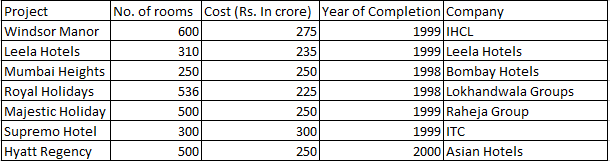### CAT 1997 Question Paper Question 2

Instructions

The following table gives the number of rooms in different hotels in Mumbai and the cost of building each one of them. Note that all the projects started in 1997Note: All projects start in 1997.

Question 2

# Which of the following has the maximum number of rooms per crore of rupees?

Solution

For the above answer, the ratio of number of rooms to the cost should be maximum. It is maximum for lokhandwala group which is 536/225 = 2.38

• All Quant CAT Formulas and shortcuts PDF
• 30+ CAT previous papers with solutions PDF